Short & Long Answer Questions (part- 2)- Force and Laws of Motion

# Short & Long Answer Questions (part- 2)- Force and Laws of Motion - Science Class 9

Ques 1: Explain Newton’s second law of motion and with the-help of an example show how it is used in sports.

Ans: Newton’s second law of motion: The rate of change of momentum of an object is proportional to the applied unbalanced force in the direction of the force.

Let us assume:

Object of mass m, is moving along a straight line with an initial velocity ‘u’, It is uniformly accelerated to velocity v in time ‘t by the application of force,

F throughout the time 't'.

Initial momentum of the object = p1 = mu

Final momentum of the object = p2 = mv

The change in momentum ∝ p2-p1

∝ mv-mu

= m (v-u)

The rate of change of momentum ∝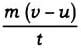∴ Applied force F ∝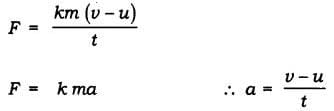K = constant of proportionality

F = kg m/s2 = Newton

Use of second law of motion in sports :

In cricket field, the fielder gradually pulls his hands backward while catching a ball. The fielder catches the ball and gives swing to his hand to increase the time during which the high velocity of the moving ball decreases to zero.The acceleration of the ball is decreased and therefore the impact of catching the fast moving balls reduced.

If not done so, then the fast moving ball will exert large force and may hurt the fielder.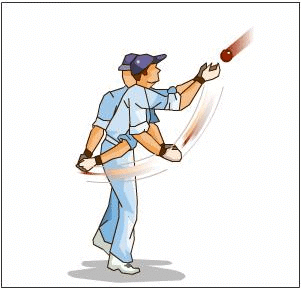Ques 2: State all 3 Newton’s law of motion. Explain inertia and momentum.

Ans: Newton’s I law of motion: An object remains in a state of rest or of uniform motion in a straight line unless acted upon by an external unbalanced force.

Newton’s II law of motion: The rate of change of momentum of an object is proportional to the applied unbalanced force in the direction of the-force.

Newton’s III law of motion: To every action, there is an equal and opposite reaction and they act on two different bodies.

Inertia: The natural tendency of an object to resist a change in their state of rest or of uniform motion is called inertia.

Momentum: The momentum of an object is the product of its mass and velocity and has the same direction as that of the velocity. Its S.I. unit is kgm/s. p = m x v

Ques 3: Define force. Give its unit and define it. What are different types forces?

Ans: Force: It is a push or pull on an object that produces acceleration in the body on which it acts.

A force can do 3 things on a body

(a) It can change the speed of a body.

(b) It can change the direction of motion of a body.

(c) It can change the shape of the body.

The S.I. unit of force is Newton.

Newton: A force of one Newton produces an acceleration of 1 m/s2 on an object of mass 1 kg.

1N = 1kg m/s2

Types of forces:

(i) Balanced force: When the forces acting on a body from the opposite direction do not change the state of rest or of motion of an object, such forces are called balanced forces.

(ii)Unbalanced force: When two opposite forces acting on a body move a body in the direction of the greater force or change the state of rest, such forces are called as unbalanced force.

(ii)Frictional force: The force that always opposes the motion of object is called force of friction.

Ques 4: What is inertia? Explain different types of inertia. Give 3 examples in daily life which shows inertia.

Ans: Inertia: The natural tendency of an object to resist change in their state of rest or of motion is called inertia.

The mass of an object is a measure of its inertia. Its S.I. unit is kg.

Types of inertia:

Inertia of rest: The object at rest will continue to remain at rest unless acted upon by an external unbalanced force.

Inertia of motion: The object in the state of uniform motion will continue to remain in motion with same speed and direction unless it is acted upon by an external unbalanced force. .

Three examples of inertia in daily life are:

1. When we are travelling in a vehicle and sudden brakes are .applied we tend to fall forward.

2. When we shake the branch of a tree vigorously, leaves fall down.

3. If we want to remove the dust from carpet we beat the carpet so that dust fall down.

## Activity-based Questions

Ques 1: • Make a pile of similar carom coins on a table, as shown in the figure.

• Attempt a sharp horizontal hit at the bottom of the pile using another carom coin or striker. If the hit is strong enough the bottom coin moves out quickly. Once the lowest coin is removed, the inertia of the other coins makes them ‘fall’ vertically on the table.

Inertia: It is the tendency of a body to maintain its state of rest or of motion.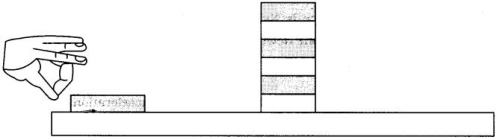Ques 2: • Set a five-rupees coin on a stiff card covering an empty glass tumbler standing on a table as shown in the figure.

• Give the card a sharp horizontal flick with a finger. If we do it fast then the card shoots away, allowing the coin to fall vertically into the glass tumbler due to its inertia.

• The inertia of the coin tries to maintain its state of rest even when the card flows off.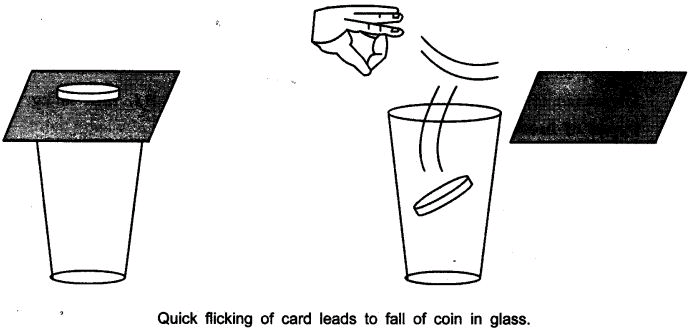Ans: The force applied on the card due to flicking changes the inertia of the card but the coin resist a change and stay at the rest i.e. inertia of rest and due to gravity falls down in the tumbler.

Ques 3: Place a water-filled tumbler on a tray.

• Hold the tray and turn around as fast as you can.

• We observe that the water spills. Why?

Ans: The water-filled in tumbler and tray are at rest. On moving/turning around the tray at faster speed the water spills because the tray and the tumbler comes into motion while the water in the tumbler remain at inertia of rest.

Ques 4:• Request two children to stand on two separate carts as shown on the next page.

• Give them a bag full of sand or some other heavy object. Ask them to play a game of catch with the bag.

• Does each of them receive an instantaneous reaction as a result of throwing the sand bag (action)?

• You can paint a white line on cartwheels to observe the motion of the two carts when the children throw the bag towards each other.

Ans: Yes, in this case each of them receives an instantaneous reaction as a result of throwing the sand bag.

This activity explain Newton’s III law of motion i.e., the force is exerted forward in throwing the bag full of sand and the person who is throwing it gets pushed backward.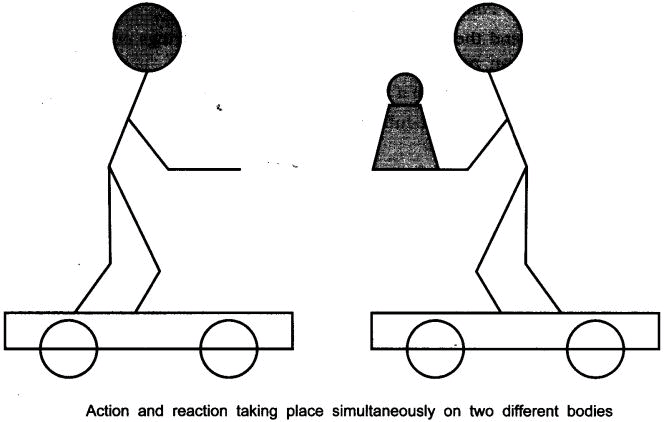Ques 5: • Take a big rubber balloon and inflate it fully. Tie its neck using a thread. Also using adhesive tape, fix a straw on the surface of this balloon.
• Pass a thread through the straw and hold one end of the thread in your hand or fix it on the wall.
• Ask your friend to hold the other end of the thread or fix it on a wall at some distance. The arrangement is shown in the figure below.
• Now remove the thread tied on the neck of balloon. Let the air escape from the mouth of the balloon.
• Observe the direction in which the straw moves.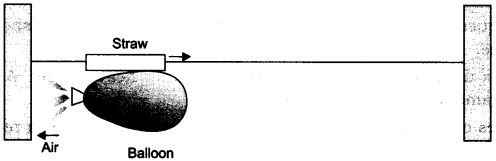Observation:
When the air escapes out from the balloon the straw moves in the opposite direction of the air moved out of the balloon.
This activity explains the law of conservation of momentum and Newton’s III law of motion.
Initial momentum = Final momentum

Ques 6: • Take a test tube of good quality glass material and put a small amount of water in it. Place a stop cork at the mouth of it.
• Now suspend the test tube horizontally by two strings or wires as shown in the figure on next page.
• Heat the test tube with a burner until water vaporises and the cork blows out.
• Observe that the test tube recoils in the direction opposite to the direction of the cork.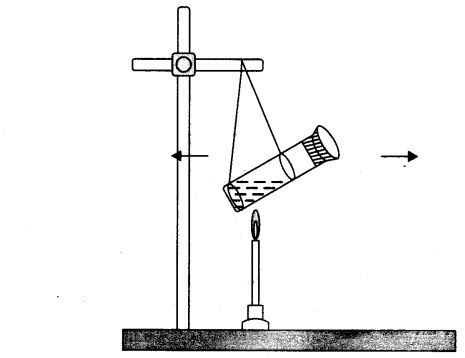Observation:
The cork is pushed out in forward direction by the hot steam. The test tube is pushed in the backward direction.
It explain Newton’s III law of motion and conservation of momentum.

## Value-based Questions

Ques 1: Class V students were playing cricket with the cork hall in the school campus. Charu a senior student told them about the accidents that can occur due to cork ball in the campus and also advised them to bring soft cosco ball to play the game.

(a) Why it was safe to play with soft ball and not with hard cork ball?

(b) A player pulls his hands backwards after holding the ball shot at high speed. Why?

(c) What value of Charu is seen in this act?

Ans: (a) The soft ball will have less inertia as compared to the heavy ball and it would not hurt the players.

(b) By pulling the hand backwards it reduces the force exerted by the ball on hands.

(c) Charu showed the value of being responsible and helpful by nature.

Ques 2: Saksham saw his karate expert friend breaking a slate. He tried to break the slate but Saksham’s friend stopped him from doing so and told him that it would hurt, one needs lot of practice in doing so.

(a) How can a karate expert break the slate without any injury to his hand?

(b) What is Newton’s third law of motion?

(c) What value of Saksham’s friend, is seen in the above case?

Ans: (a) A karate player applies the blow with large velocity in a very short interval of time on the slate, therefore large force is exerted on the slate and it breaks.

(b) To every action there is an equal and opposite reaction, both act on different bodies.

Saksham’s friend showed the value of being responsible and caring friend.

The document Short & Long Answer Questions (part- 2)- Force and Laws of Motion | Science Class 9 is a part of the Class 9 Course Science Class 9.
All you need of Class 9 at this link: Class 9

## Science Class 9

66 videos|352 docs|97 tests

## FAQs on Short & Long Answer Questions (part- 2)- Force and Laws of Motion - Science Class 9

 1. What are the three laws of motion?Ans. The three laws of motion are: 1. Newton's First Law of Motion: Also known as the law of inertia, it states that an object at rest will remain at rest, and an object in motion will continue moving in a straight line at a constant speed, unless acted upon by an external force. 2. Newton's Second Law of Motion: It states that the force acting on an object is equal to the mass of the object multiplied by its acceleration. This law can be mathematically expressed as F = ma, where F is the force, m is the mass, and a is the acceleration. 3. Newton's Third Law of Motion: It states that for every action, there is an equal and opposite reaction. This means that whenever an object exerts a force on another object, the second object exerts an equal and opposite force on the first object.
 2. What is the difference between mass and weight?Ans. Mass and weight are two different properties of an object. The key differences between them are: - Mass: Mass is the amount of matter present in an object. It is a scalar quantity and is measured in kilograms (kg). Mass remains the same regardless of the location of the object. In other words, an object will have the same mass on Earth as it would on the Moon or any other planet. - Weight: Weight is the force exerted by gravity on an object. It is a vector quantity and is measured in newtons (N). Weight depends on the mass of the object as well as the gravitational pull acting on it. Therefore, an object will have different weights on different celestial bodies due to variations in gravity.
 3. How does Newton's first law of motion apply to everyday life?Ans. Newton's first law of motion, also known as the law of inertia, can be observed in several everyday life situations. Some examples include: - A book on a table: When a book is placed on a table and left undisturbed, it remains at rest until an external force, such as someone pushing it, is applied. - A car coming to a stop: When a car is moving and the brakes are applied, the car comes to a stop because of the friction between the tires and the road. Without friction, the car would continue moving in a straight line at a constant speed. - A ball rolling on a level ground: If a ball is rolling on a level ground and there is no external force acting on it, it will continue rolling with the same speed and direction.
 4. How does Newton's second law of motion relate force, mass, and acceleration?Ans. Newton's second law of motion states that the force acting on an object is directly proportional to its mass and acceleration. Mathematically, this can be expressed as F = ma, where F is the force, m is the mass of the object, and a is its acceleration. This law essentially means that the force required to accelerate an object is directly proportional to its mass. In other words, the larger the mass of an object, the greater the force required to accelerate it. Similarly, the greater the acceleration of an object, the greater the force required to achieve that acceleration.
 5. Can you provide an example of Newton's third law of motion?Ans. Yes, an example of Newton's third law of motion is the action-reaction forces experienced when a person jumps off a boat onto a dock. When a person jumps off a boat onto a dock, they exert a force on the dock in the forward direction. According to Newton's third law, the dock simultaneously exerts an equal and opposite force on the person in the backward direction. This force pushes the person back onto the boat, preventing them from falling into the water. This example illustrates how every action has an equal and opposite reaction, as the person's action of exerting a force on the dock results in the dock exerting an equal and opposite force on the person.

## Science Class 9

66 videos|352 docs|97 testsExplore Courses for Class 9 examSignup to see your scores go up within 7 days! Learn & Practice with 1000+ FREE Notes, Videos & Tests.
10M+ students study on EduRev
Track your progress, build streaks, highlight & save important lessons and more!
Related Searches

,

,

,

,

,

,

,

,

,

,

,

,

,

,

,

,

,

,

,

,

,

;